• 将两个二维数组相乘应该怎么计算？有没有可以实现二维数组乘法运算的函数思路？
• 之前看到有人在网上询问如何用Java多多线程实现二维数组的乘法。于是回去仔细思考了一下，实现出来分享一下。

之前看到有人在网上询问如何用Java多多线程实现二维数组的乘法。于是回去仔细思考了一下，实现出来分享一下。
首选我使用的是Runnable接口。将乘法运算部分放入run()中。

package com.thread.arr;

public class ArrThread implements Runnable {
public void run() {
// TODO Auto-generated method stub
int x = p.x;
int y = p.y;
//System.out.println(x+","+y);
}
}
}
}
主函数类如下：每个线程计算结果数组中的一个值。

package com.thread.arr;

import java.io.ObjectInputStream.GetField;

static int m;
static int n;
static int l;
private static Position p;
private static int LEN = 4;
static int Arra[][];
static int Arrb[][];
static int Arrc[][];

private static void fillArr(int Arr[][]){
for(int i=0; i<Arr.length; i++)
for(int j=0; j<Arr[i].length; j++){
Arr[i][j] = i+j;
}
}

private static void printArr(int Arr[][]){
for(int i=0; i<Arr.length; i++){
for(int j=0; j<Arr[i].length; j++){
System.out.print(Arr[i][j]+"  ");
}
System.out.println("");
}
}

public static synchronized Position getPosition(){
p.y++;
if(p.y>=l){
p.x++;
p.y=0;
}
return p;
}

public static void SingleThread(int[][] a, int[][] b, int[][] c){
for(int i=0; i<c.length; i++)
for(int j=0; j<c[i].length; j++){
for(int k=0; k<b.length; k++)
c[i][j]+= a[i][k]*b[k][j];
}
}

public static void main(String[] args) throws InterruptedException {
m = 2;
n = 3;
l = 4;
p = new Position();
Arra = new int[m][n];
Arrb = new int[n][l];
Arrc = new int[m][l];
fillArr(Arra);
fillArr(Arrb);
printArr(Arra);
printArr(Arrb);
for(int i=0; i<m*l; i++){
t.start();
}

printArr(Arrc);
}
}
还增加了一个Position类，作为同步的位置信息类

package com.thread.arr;

public class Position {
int x;
int y;

public Position() {
// TODO Auto-generated constructor stub
this.x = 0;
this.y = -1;
}

}


展开全文Java 多线程
• ## 二维数组 矩阵相乘

千次阅读 2016-12-08 21:22:17
两个矩阵相乘，可以用3个二维数组解决。两个存初始值，一个存结果。 #include using namespace std; #define rows 8 #define cols 8 int a[rows][cols]; int b[rows][cols]; int c[rows][cols]; int main() { int ...
两个矩阵相乘，可以用3个二维数组解决。两个存初始值，一个存结果。


#include<iostream>
using namespace std;
#define rows 8
#define cols 8
int a[rows][cols];
int b[rows][cols];
int c[rows][cols];
int main()
{
int m,n,i,j,k,p,q;
cin>>m>>n;//输入矩阵a
for(i=0;i<m;++i)
for(j=0;j<n;++j)
cin>>a[i][j];

cin>>p>>q;//输入矩阵b
for(i=0;i<p;++i)
for(j=0;j<q;++j)
cin>>b[i][j];

for(i=0;i<m;++i){//矩阵c=a*b
for(j=0;j<q;++j){
c[i][j]=0;
for(k=0;k<n;++k)
c[i][j]+=a[i][k]*b[k][j];
}
}

for(i=0;i<m;++i){//输出矩阵c
for(j=0;j<q;++j)
{
cout<<c[i][j]<<" ";
}
cout<<endl;
}
system("pause");
return 0;
}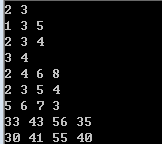展开全文• 1 //两个二维数组相乘 2 #include<stdio.h> 3 #include<stdlib.h> 4 #define M 2 5 #define N 3 6 //#define P 6 7 void main() 8 { 9 int i,j,k; 10 //二维数组A和B求乘积，积放在...


1 //两个二维数组相乘
2 #include<stdio.h>
3 #include<stdlib.h>
4 #define M 2
5 #define N 3
6 //#define P 6
7 void main()
8 {
9     int i,j,k;
10     //二维数组A和B求乘积，积放在数组C中
11     int A[M][N],B[N][M],C[M][M];
12     //输入二维数组A的元素的值
13     printf("请输入矩阵A的元素：");
14     for(i=0;i<M;i++)
15     {
16         for(j=0;j<N;j++)
17         {
18             scanf("%d",&A[i][j]);
19         }
20     }
21     printf("\n");
22     //打印二维数组A的元素
23     printf("矩阵A如下：\n");
24     for(i=0;i<M;i++)
25     {
26         for(j=0;j<N;j++)
27         {
28             printf("%3d",A[i][j]);
29         }
30         printf("\n");
31     }
32     printf("\n");
33     //输入二维数组B的元素的值
34     printf("请输入矩阵B的元素：");
35     for(i=0;i<N;i++)
36     {
37         for(j=0;j<M;j++)
38         {
39             scanf("%d",&B[i][j]);
40         }
41     }
42     printf("\n");
43     //打印二维数组B的元素
44     printf("矩阵B如下：\n");
45     for(i=0;i<N;i++)
46     {
47         for(j=0;j<M;j++)
48         {
49             printf("%3d",B[i][j]);
50         }
51         printf("\n");
52     }
53     //数组相乘
54     printf("\n矩阵A乘以矩阵B结果为：\n");
55     for(i=0;i<M;i++)
56     {
57         for(j=0;j<M;j++)
58         {
59             C[i][j] = 0;
60             for(k=0;k<N;k++)
61                 C[i][j] = C[i][j]+A[i][k]*B[k][j];
62         }
63     }
64     printf("\n");
65     //数组C中的值分行显示
66     for(i=0;i<M;i++)
67     {
68         for(j=0;j<M;j++)
69         {
70             printf("%3d",C[i][j]);
71         }
72         printf("\n");
73     }
74     system("pause");
75 }转载于:https://www.cnblogs.com/sun-/p/5022107.html
展开全文• 二维数组 就是一个由行和列组成的一个表格而已，矩阵Matrix。 我们在这个矩阵中访问元素的话，是根据元素的行角标和列角标所确定的。 1、那么对于二维数组是如何存储的呢？ 无论是二维数组，还是多维数组，...
二维数组

就是一个由行和列组成的一个表格而已，矩阵Matrix。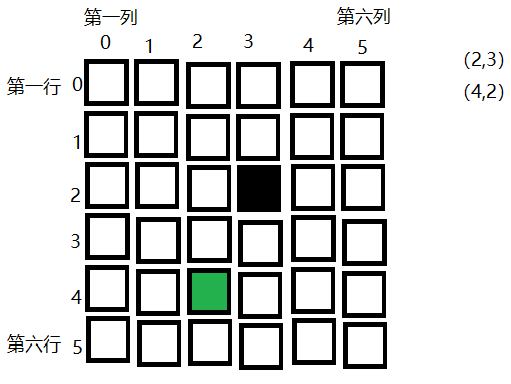我们在这个矩阵中访问元素的话，是根据元素的行角标和列角标所确定的。
1、那么对于二维数组是如何存储的呢？
无论是二维数组，还是多维数组，它们本身就是一个一维数组；尤其对于二维数组而言，无非就是一个一维数组，只不过该一维数组中的每一个元素是另一个一维数组罢了！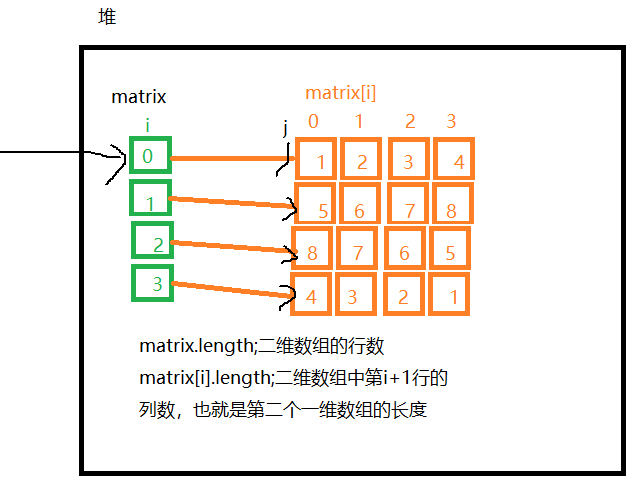int[][] matrix=new int[m][n];
内存中一共有m+1个一维数组
class Test01{
public static void main(String[] args){
int[][] matrix={
{1,2,3,4},
{5,6,7,8},
{8,7,6,5},
{4,3,2,1}
};
//matrix.length 表示的就是最外层那个一维数组的长度 行数
//matrix[i] 表示的是最外层那个一维数组当中角标i的那个元素 只不过这个元素也是一个一维数组
//matrix[i].length 表示的是这个一维数组元素的长度(当前行的长度)
//matrix[i][j] 表示的就是这个一维数组元素中角标j的那个元素
for(int i=0;i<matrix.length;i++){
for(int j=0;j<matrix[i].length;j++){
System.out.print(matrix[i][j]+" ");
}
System.out.println();
}
}
}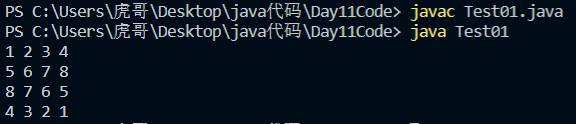既然以上二维数组的遍历（打印）已经出现，那么现在我们再来讲一讲如何创建二维数组：
1.int[][]  matrix=new int;//创建一个3行4列的二维数组，元素默认都是0
创建个指定元素的二维数组（3种方式都可以）
2.int[][] matrix=new int[][]{
{1,2,3,4} ,
{5,6,7,8} ,
{9,10,11,12}
};
___________________
3.int[][] matrix={
{1,2,3,4} ,
{5,6,7,8} ,
{9,10,11,12}
};
____________________
锯齿矩阵/二维数组
int[][] matrix={
{1,2,3,4} ,
{5,6,7} ,
{8,9},
{10}
};
注意：特别的，如果一个二维数组的行和列相等的话，也称之为是方阵。矩阵一定是二维数组，二维数组不一定是矩阵。
二维数组典例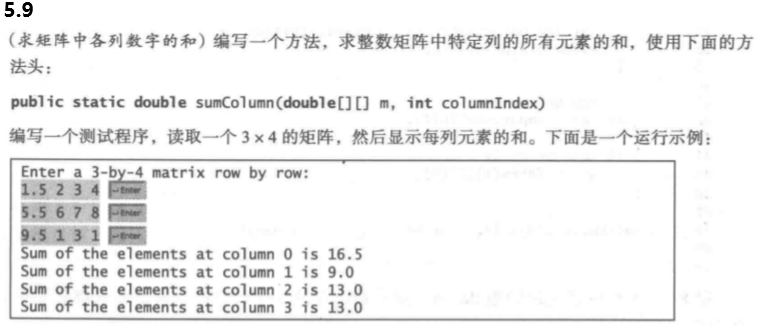import java.util.*;
class Demo05_09{
public static void main(String[] args){
/*
(0,0) (0,1) (0,2) (0,3)
(1,0) (1,1) (1,2) (1,3)
(2,0) (2,1) (2,2) (2,3)
累加行的时候 行不动 列动
累加列的时候 列不动 行动
*/
//1.输入一个3*4的矩阵
Scanner scanner=new Scanner(System.in);
System.out.println("Enter numbers:");
double[][] matrix=new double;
for(int i=0;i<matrix.length;i++){
for(int j=0;j<matrix[i].length;j++){
matrix[i][j]=scanner.nextDouble();
}
}
//2.打印每列的和
for(int col=0;col<matrix.length;col++){
System.out.println(sumColumn(matrix,col));
}
}
public static double sumColumn(double[][] m,int col){
double sum=0;
for(int row=0;row<m.length;row++){
sum+=m[row][col];
}
return sum;
}
}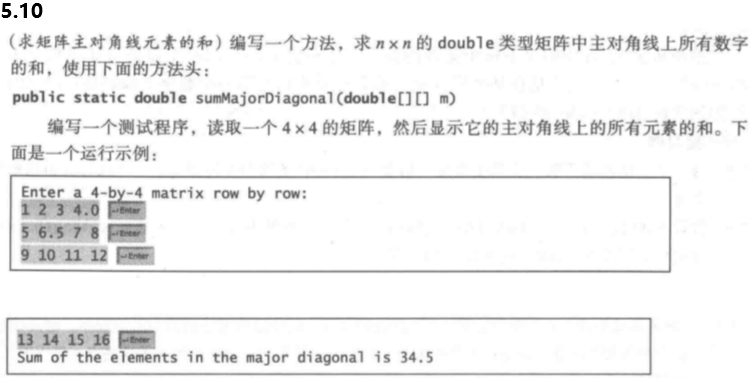class Demo05_10{
public static void main(String[] args){
/*
(0,0) (0,1) (0,2)
(1,0) (1,1) (1,2)
(2,0) (2,1) (2,2)
主对角线上的元素 行列角标是相等的
*/
int[][] m={
{1, 2, 3, 4},
{5, 6, 7, 8},
{9, 10,11,12},
{13,14,15,16}
};
//主对角线
int sum=0;
for(int i=0;i<m.length;i++){
sum+=m[i][i];
}
System.out.println(sum);
//副对角线
int subsum=0;
for(int i=0;i<m.length;i++){
subsum+=m[i][m.length-1-i];
}
System.out.println(subsum);
/*
for(int i=0;i<m.length;i++){
for(int j=0;j<m[i].length;j++){
if(i==j){
sum+=m[i][j];
}
}
}
*/
}
}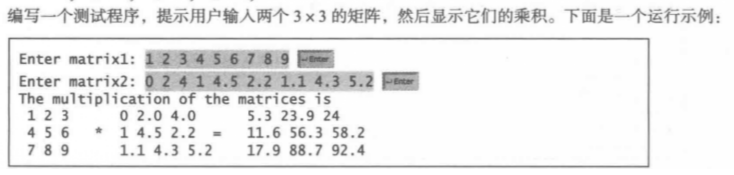class Demo05_11{
public static void main(String[] args){
//m*n n*p m*p 矩阵相乘 前者的列 必须等于 后者的行
/*
1 2 3        1 2    1*1+2*3+3*5   1*2+2*4+3*6
×    3 4  =
4 5 6        5 6    4*1+5*3+6*5   4*2+5*4+6*6
对于数学上的一些运算公式 如果直接通过看计算流程还是比较麻烦的
此时推荐使用已知公式计算！
*/
double[][] A={ //m*p
{1,2,3},
{4,5,6},
{7,8,9}
};
double[][] B={ //p*n
{0,2.0,4.0},
{1,4.5,2.2},
{1.1,4.3,5.2}
};
double[][] C=new double[A.length][B.length];//m*n
for(int i=0;i<C.length;i++){
for(int j=0;j<C[i].length;j++){
double sum=0;
for(int k=0;k<B.length;k++){
sum+=A[i][k]*B[k][j];
}
C[i][j]=sum;
System.out.print(C[i][j]+"　");
}
System.out.println();
}
}
}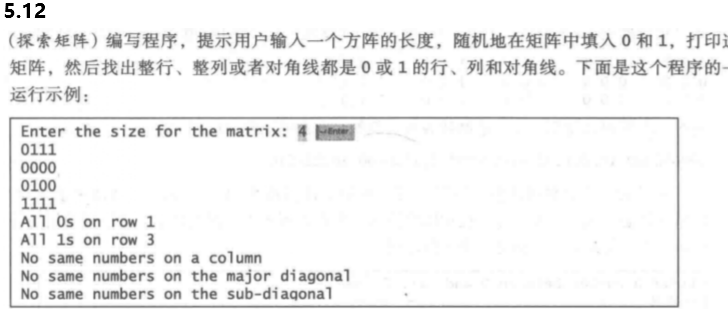import java.util.*;
class Demo05_12{
public static void main(String[] args){
//1.输入方针的尺寸 创建方阵
Scanner scanner=new Scanner(System.in);
System.out.print("Enter size:");
int size=scanner.nextInt();
int[][] m=new int[size][size];
//2.随机的给方阵中填入0或1
Random random=new Random();
for(int i=0;i<size;i++){
for(int j=0;j<size;j++){
m[i][j]=random.nextInt(2);
System.out.print(m[i][j]+" ");
}
System.out.println();
}
//3.让行，列，对角线累加 sum==0 sum==size 表示全相等
checkRow(m);
checkCol(m);
checkDiagonal(m);
checkSubDiagonal(m);
}
public static void checkSubDiagonal(int[][] m){
int sum=0;
for(int i=0;i<m.length;i++){
sum+=m[i][m.length-1-i];
}
if(sum==m.length||sum==0){
System.out.printf("副主对角线全相等且是%d\n",sum==0?0:1);
}
}
public static void checkDiagonal(int[][] m){
int sum=0;
for(int i=0;i<m.length;i++){
sum+=m[i][i];
}
if(sum==m.length||sum==0){
System.out.printf("主对角线全相等且是%d\n",sum==0?0:1);
}
}
public static void checkRow(int[][] m){
for(int i=0;i<m.length;i++){
int sum=0;
for(int j=0;j<m[i].length;j++){
sum+=m[i][j];
}
if(sum==m.length||sum==0){
System.out.printf("第%d行全相等且是%d\n",i+1,sum==0?0:1);
}
}
}
public static void checkCol(int[][] m){
for(int j=0;j<m.length;j++){
int sum=0;
for(int i=0;i<m.length;i++){
sum+=m[i][j];
}
if(sum==m.length||sum==0){
System.out.printf("第%d列全相等且是%d\n",j+1,sum==0?0:1);
}
}
}
}
连续相等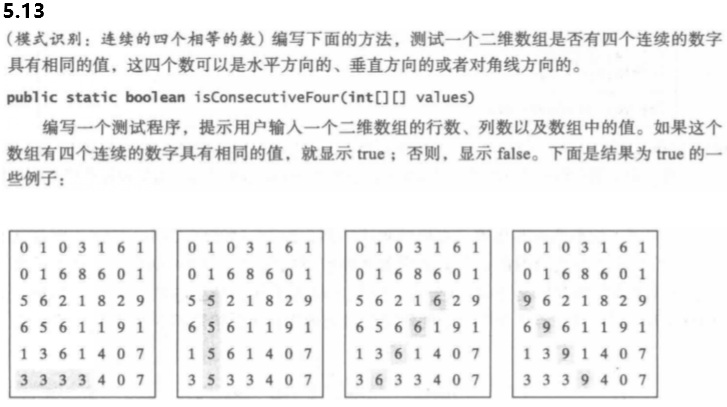对于这中连续相等的问题（本来有8个方向，但有一半会重复，所以用四个方向来考虑）：首先先画图分析、找出每个方向上满足（角标不越界）的最大角标。例如这道题：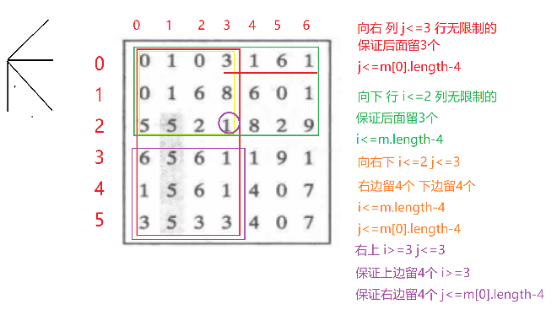import java.util.*;
class Demo05_13{
public static void main(String[] args){
Scanner scanner=new Scanner(System.in);
System.out.print("Enter row ,col:");
int row=scanner.nextInt();
int col=scanner.nextInt();
int[][] m=new int[row][col];
for(int i=0;i<row;i++){
for(int j=0;j<col;j++){
m[i][j]=scanner.nextInt();
}
}
System.out.println(isConsecutiveFour(m));
}
public static boolean isConsecutiveFour(int[][] m){
for(int i=0;i<m.length;i++){
for(int j=0;j<m[i].length;j++){
//向右
if(j<=m[i].length-4){
boolean flag=true;
for(int c=j+1;c<=j+3;c++){
if(m[i][j]!=m[i][c]){
flag=false;
break;
}
}
if(flag){
return true;
}
}
//向下
if(i<=m.length-4){
boolean flag=true;
for(int r=i+1;r<=i+3;r++){
if(m[i][j]!=m[r][j]){
flag=false;
break;
}
}
if(flag){
return true;
}
}
//向右下
if(i<=m.length-4&&j<=m.length-4){
boolean flag=true;
for(int r=i+1,c=j+1;r<=i+3;r++,c++){
if(m[i][j]!=m[r][c]){
flag=false;
break;
}
}
if(flag){
return true;
}
}
//向右上
if(i>=3&&j<=m.length-4){
boolean flag=true;
for(int r=i-1,c=j+1;c<=j+3;r--,c++){
if(m[i][j]!=m[r][c]){
flag=false;
break;
}
}
if(flag){
return true;
}
}
}
}
return false;//四个方向都没有连续的
}
}


展开全文• 分别定义两个二维数组用来存放两个矩阵，矩阵A的行号和矩阵B的列号决定矩阵C的行列号；矩阵A的列号和矩阵B的行号相同者进行乘法操作。 现在暂且不考虑设置矩阵相乘的规则，就弄个最简单的矩阵相乘 package juzhen...java 线性代数 矩阵
• 二维数组怎样才能按列的顺序转成一维数组啊 是按列的，不是按顺序有问必答 c语言
• 给定 2 个 3D 数组 A 和 B，其中 A(:,:,i) 和 B(:,:,i) 是 i 个 2D 矩阵，乘以 A(:,:,i)*B(:,:,i)不使用 for 循环。 与其说是一段实际有用的代码，不如说是编码实验，其目标是推导出一种有效的方法来将许多矩阵相乘...matlab
• } public static int[][] matrixMultiply(int[][] arrOne,int[][] arrTwo){ //创建m*p二维数组保存相乘结果 int[][] result = new int[arrOne.length][arrTwo.length]; //核心：i的范围arrOne的length，j的范围...
• 前面我们学习了一位数组，在讲解到八皇后的问题时，我们也用到了二位数组，那么什么是二维数组呢？相信大家都应该学过二维矩阵如： 其实二维数组就是一个二维的矩阵，矩阵的行列对应的位置就是二维数组每个元素...矩阵的转置
• c语言动态二维数组实现矩阵相乘 如何申请动态二维数组,代码如下 该方法是最灵活的，可以任意指定行数和列数 /* *申请a数组的内存* */ /*先申请每一行指针的内存*/ a = (int **)malloc(sizeof(int *)*a_row)...c语言 malloc sizeof
• php如何将二维数组变为一维数组 $arr = [ ['id' => 1001, 'name' => 'gsj1'], ['id' => 1002, 'name' => 'gsj2'], ['id' => 1003, 'name' => 'gsj3'], ]; //php如何将二维数组变为一维数组$...
• 二维数组转化成一维相乘的这一步不太明白 { int index1 = i * pMat1->nCol + k; //计算index1，不懂可以画个图 int index2 = k * pMat2->nCol + j; //计算index2，不懂可以画个图 sum += pMat1->pData...c++ c语言
• 近来在搞一个改进近场music算法的仿真问题，用matlab进行二维搜索的时候写了很多for循环，导致运行速度极慢。考虑到matlab语言本身的矩阵运算特点，改用三维数据解决二维搜索问题，下面记录过程中遇到的一点问题与...matlab matlab加速
•python numpy
• 二维数组 二维数组简单来说就是一个由行和列组成的一个表格，也就是数学中的矩阵。我们在这个矩阵中访问元素的话，是根据元素的行角标和列角标所确定的。 那么具体是在内存中如何存储的呢？ 无论是二维数组，...
• 若A为NxM阶矩阵 B为MxP阶矩阵，二者相乘之后的矩阵则为NXP阶矩阵 运算过程： 思路 计算并显示出来这个结果 我们则需要 一个计算方法 和 一个显示方法 因此主函数可以写的相当之简单 ， 键入想要求解的矩阵的数值 ...算法 java 线性代数 矩阵
• //二维数组存储矩阵乘法 void multi(int a, int b){ for (int i=0;i;i++) //i代表a矩阵的行数 { for (int j=0;j;j++) //j代表b矩阵的列数 { for (int k=c
• <br />/**  *两个二维数组的想相乘；  *这个两个二维数组要求行数，类型相等  */ public class Exercise6_25 {    static int[][] arr ;    public static ...编程 string class
• 实例十六：二维数组的应用（矩阵相乘） 问题描述： 编写矩阵A与矩阵B的乘积矩阵C。 /*实例十六：二维数组的应用（矩阵相乘）*/ #include<stdio.h> #define N 4 #define M 5 #define K 3 int ...
• 那么，接下来，我们将会通过对Python二维数组的理解来为大家解读这方面的知识。Python中没有数组的数据结构，但列表很像数组，如：a=[0,1,2]这时a=0, a=1, a[=2，但引出一个问题，即如果数组a想定义为0到...
• 二维数组就是在一位数组上多加了维度，把一位数组比作直线，那么二维数组就是面，由行和列组成的表格，也可以把它看成一个矩阵，所以访问二维数组的方式就必须访问行角标，列角标来具体定位。 具体来说一下二维数组...
• /*根据输入的参数，定义待乘的两个二维数组a和b，以及相乘结果矩阵re*/ for(i=0;i;i++) for(j=0;j;j++) a[i][j] = rand() % 100 + 1; for(i=0;i;i++) for(j=0;j;j++) b[i][j] = rand() % 100 + 1; /*由于并没有对...
• 有关二维数组类 需要用指向指针的指针，要不然[]操作符重载没办法操作 在new分配内存的时候，语法如下：` ...另外sizeof方面，在memset或memcpy的时候，一定要维数和int类型相乘，和二维数组不一样的是，这里的内...指向指针的指针
• 1.二维数组的初始化 二维数组的实质：一维数组的数组。 先创建一维数组，再在一维数组中存储一维数组的引用变量。 ①二维数组的初始化格式1： 数据类型[ ][ ] 变量名 = new 数据类型[m][n]; m代表二维数组中一...java
• 两个矩阵相乘 #include<stdio.h> const int N=3; const int K=2; const int M=3; int main() { int i,j,t=0;... printf("请输入二维数组a:"); for(i = 0; i < N; i++) { ...
• java 二维数组矩阵乘法的实现方法，需要的朋友可以参考一下
• 1 创建二维数组 array_1 = np.ones([10,10]) #创建一个10行10列填充值为1的二维数组 array_0 = no.zeros([3,5]) #创建一个3行5列填充值为0的二维数组 2 对二维数组中的一个元素进行操作 array_1[i,j] += 5 #...
• 二维数组 二维数组简单来说就是一个由行和列组成的一个表格而已，通俗的说就是一个矩阵Matrix。 我们在这个矩阵中访问元素的话，是根据元素的行角标和列角标所确定的。 二维数组具体是在内存中如何存储的呢？ ......